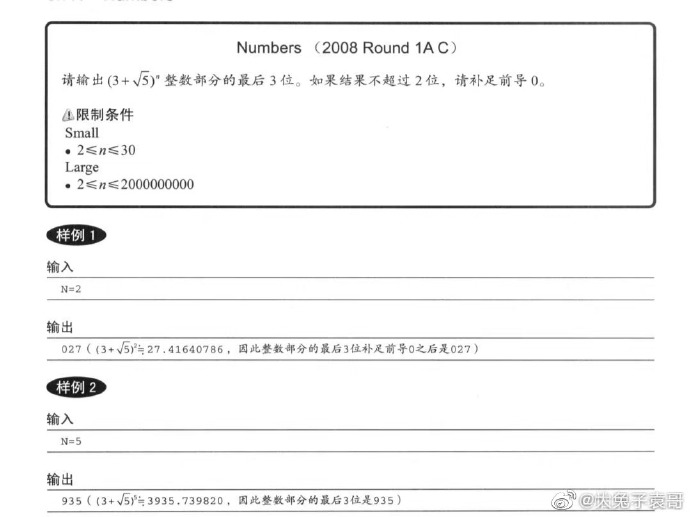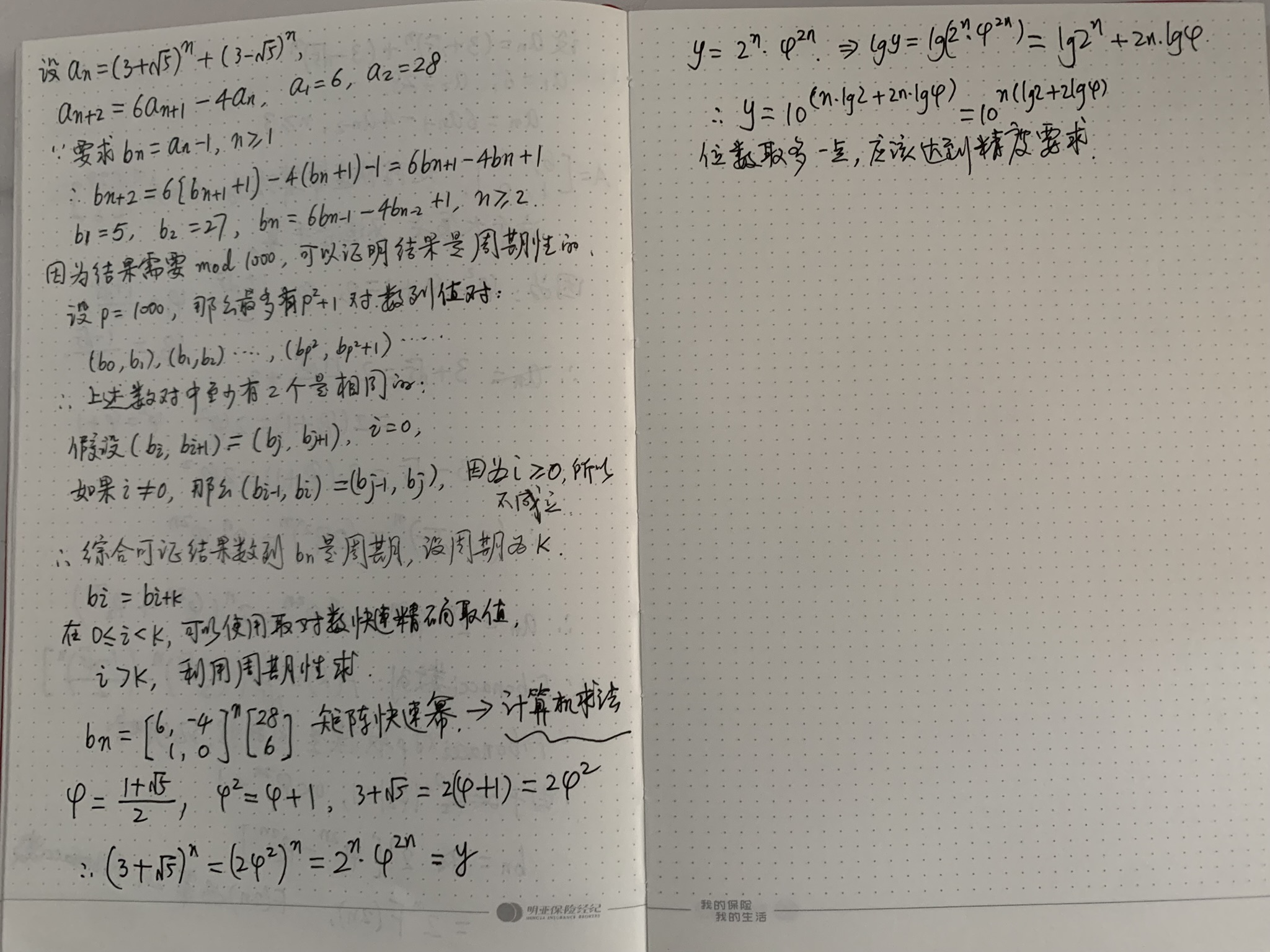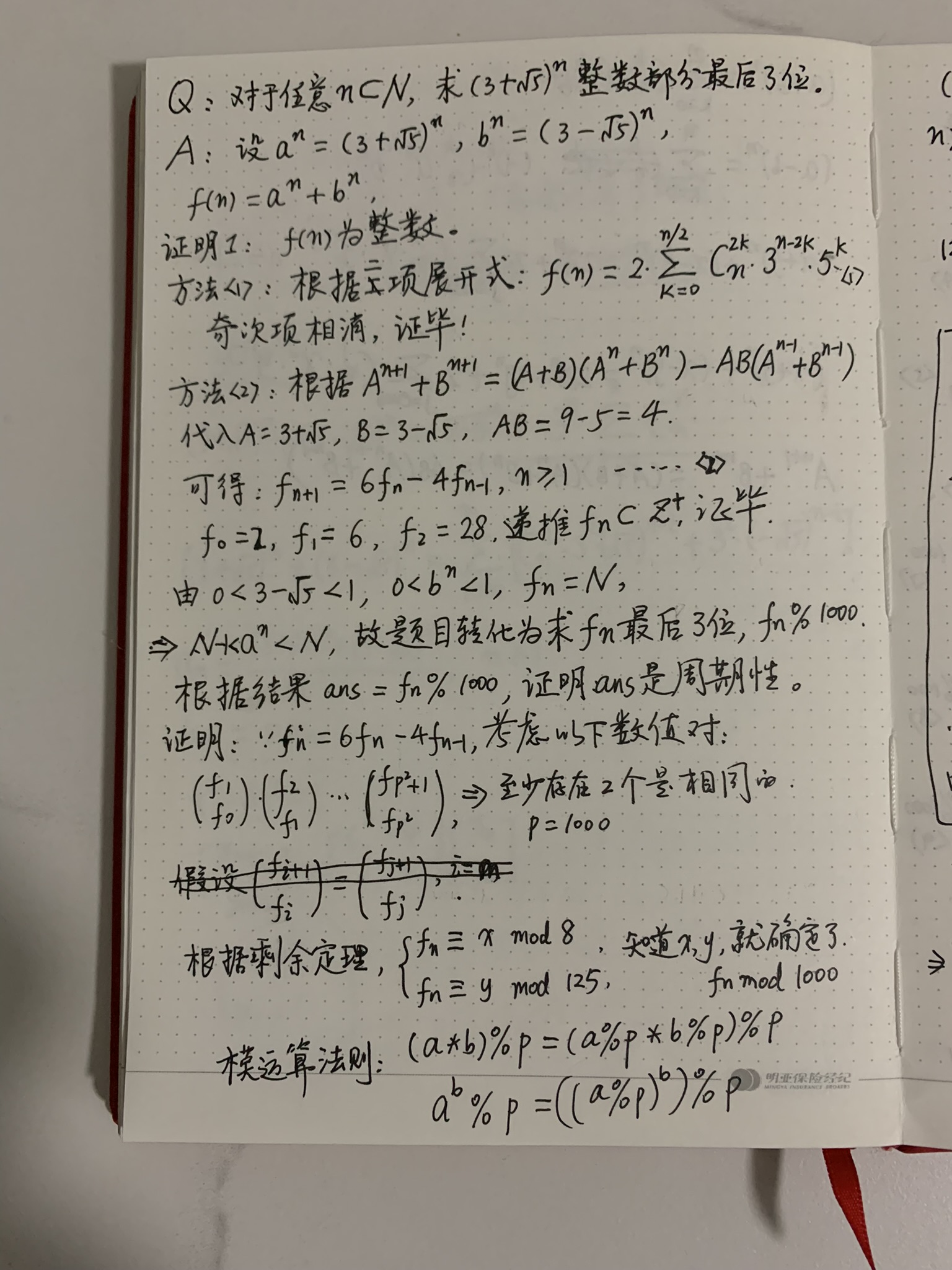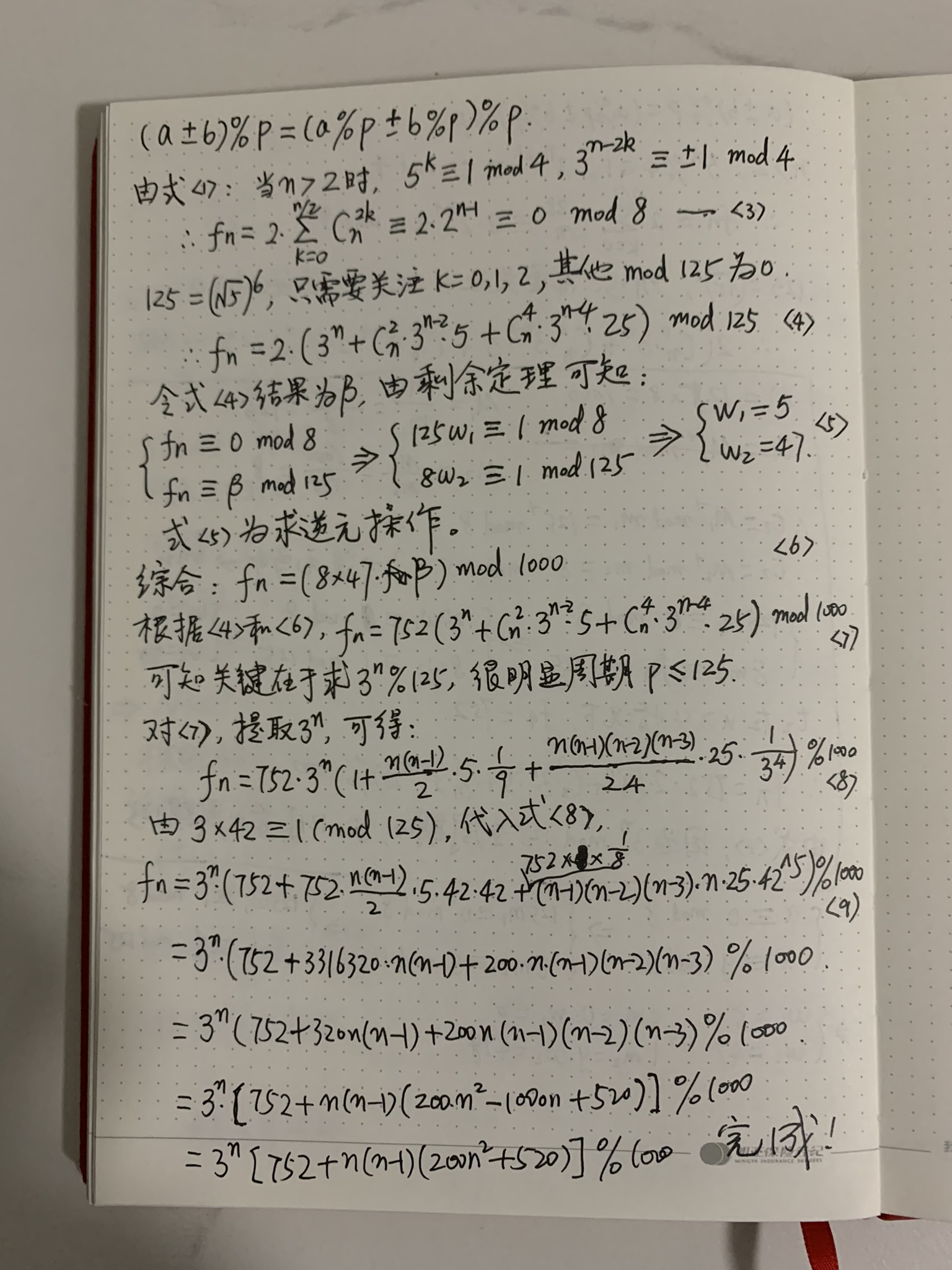By Long Luo$y = (3+ \sqrt{5})^n$ ，两边同取对数，$\log_{10}{y} = n \log_{10}{(3+\sqrt5)}$$y = 10^{n\log_{10}{5.23607}}$$lg5 \approx 0.7$ ，所以 $y \approx 10^{0.7n}$Problem:
In this problem, you have to find the last three digits before the decimal point for the number $(3 + \sqrt{5})^n$ .
For example, when $n = 5$ , $(3 + \sqrt{5})^5 = 3935.73982...$ , The answer is $935$ .
For $n = 2$ , $(3 + \sqrt{5})^2 = 27.4164079...$ , The answer is $027$ .

Input:
The first line of input gives the number of cases, T. T test cases follow, each on a separate line. Each test case contains one positive integer n.

Output:
For each input case, you should output:

Case #X: Y
where X is the number of the test case and Y is the last three integer digits of the number (3 + √5)n. In case that number has fewer than three integer digits, add leading zeros so that your output contains exactly three digits.

Limits
Time limit: 30 seconds per test set.
Memory limit: 1GB.
1 ≤ T ≤ 100

Small dataset (Test set 1 - Visible)
2 ≤ n ≤ 30

Large dataset (Test set 2 - Hidden)
2 ≤ n ≤ 2000000000

$a = 3 + \sqrt{5}$ , $b = 3 - \sqrt{5}$ , 则 $f(n) = a^n + b^n$ .

$f(n) = (3 + \sqrt{5})^n + (3 - \sqrt{5})^n , n = 1, 2, \dots$